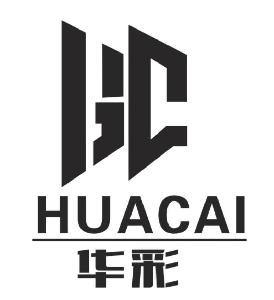20年只专注做好一件事-----做好门窗“华彩门窗”匠心打造.私人订制领导品牌。浏览量：15341

• 红星美凯龙店航拍
• 海港店航拍
• 鸿泰店航拍
• 开发区店高空航拍
• 正门
• 厂区
• 车间一
• 生产车间二
• 生产车间三
• 生产车间四
• 生产车间五
• 生产车间六
• 生产车间八
• 玻璃车间
• 接待台
• 展厅二
• 展厅三
• 展厅四
• 展厅五
• 展厅六
• 展厅七
• 展厅八
• 展厅九
• 展厅十
• 展厅十一
• 展厅十二
• 展厅十三
• 展厅十四
• 展厅十五
• 展厅正门
• 展厅一-
• 展厅二
• 展厅三
• 展厅四
• 展厅五
• 展厅六
• 展厅七
• 展厅八
• 展厅九
• 展厅十
• 展厅十一
• 展厅十二
• 展厅十三
• 展厅一
• 展厅二
• 展厅三
• 展厅四
• 展厅五
• 展厅六
• 展厅七
• 展厅八
• 海港店航拍
• 展厅九
• 开发区店低空航拍
• 展厅十
• 展厅九
• 展厅一
• 展厅二
• 展厅六
• 展厅七
• 展厅四
• 展厅三
• 展厅五
• 展厅八
• 鸿泰广场店
• 展厅正门
• 接待台
• 展厅(1)
• 展厅(2)
• 展厅(3)
• 展厅(4)
• 展厅(5)
• 展厅(6)
• 展厅(7)
• 展厅(8)
• 接待室
• 红星美凯龙店航拍
• 海港店航拍
• 鸿泰店航拍
• 开发区店高空航拍
• 正门
• 厂区
• 车间一
• 生产车间二
• 生产车间三
• 生产车间四
• 生产车间五
• 生产车间六
• 生产车间八
• 玻璃车间
• 接待台
• 展厅二
• 展厅三
• 展厅四
• 展厅五
• 展厅六
• 展厅七
• 展厅八
• 展厅九
• 展厅十
• 展厅十一
• 展厅十二
• 展厅十三
• 展厅十四
• 展厅十五
• 展厅正门
• 展厅一-
• 展厅二
• 展厅三
• 展厅四
• 展厅五
• 展厅六
• 展厅七
• 展厅八
• 展厅九
• 展厅十
• 展厅十一
• 展厅十二
• 展厅十三
• 展厅一
• 展厅二
• 展厅三
• 展厅四
• 展厅五
• 展厅六
• 展厅七
• 展厅八
• 海港店航拍
• 展厅九
• 开发区店低空航拍
• 展厅十
• 展厅九
• 展厅一
• 展厅二
• 展厅六
• 展厅七
• 展厅四
• 展厅三
• 展厅五
• 展厅八
• 鸿泰广场店
• 展厅正门
• 接待台
• 展厅(1)
• 展厅(2)
• 展厅(3)
• 展厅(4)
• 展厅(5)
• 展厅(6)
• 展厅(7)
• 展厅(8)
• 接待室

• 航拍
• 华彩门窗生产基地
• 红星美凯龙展厅
• 华彩门窗工厂展厅
• 港务局展厅
• 开发区美迎美家展厅
• 鸿泰广场店